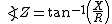xAcoustic impedanceEncyclopedia

The acoustic impedance at a particular frequency indicates how much sound pressure is generated by a given air vibration at that frequency. The acoustic impedance Z (or sound impedance) is frequency (f) dependent and is very useful, for example, for describing the behaviour of musical wind instruments. Mathematically, it is the sound pressure
Sound pressure
Sound pressure or acoustic pressure is the local pressure deviation from the ambient atmospheric pressure caused by a sound wave. Sound pressure can be measured using a microphone in air and a hydrophone in water...

p divided by the particle velocity
Particle velocity
Particle velocity is the velocity v of a particle in a medium as it transmits a wave. In many cases this is a longitudinal wave of pressure as with sound, but it can also be a transverse wave as with the vibration of a taut string....

v and the surface area S, through which an acoustic wave of frequency f propagates. If the impedance is calculated for a range of excitation frequencies the result is an impedance curve. Planar, single-frequency traveling waves have acoustic impedance equal to the characteristic impedance divided by the surface area, where the characteristic impedance is the product of longitudinal wave
Longitudinal wave
Longitudinal waves, as known as "l-waves", are waves that have the same direction of vibration as their direction of travel, which means that the movement of the medium is in the same direction as or the opposite direction to the motion of the wave. Mechanical longitudinal waves have been also...

velocity and density
Density
The mass density or density of a material is defined as its mass per unit volume. The symbol most often used for density is ρ . In some cases , density is also defined as its weight per unit volume; although, this quantity is more properly called specific weight...

of the medium. Acoustic impedance can be expressed in either its constituent units (pressure per velocity per area) or in rayl
Rayl
A rayl is one of two units of specific acoustic impedance.When sound waves pass through any physical substance the pressure of the waves causes the particles of the substance to move....

s per square meter.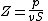Note that sometimes vS is referred to as the volume velocity.

The specific acoustic impedance z is the ratio of sound pressure
Sound pressure
Sound pressure or acoustic pressure is the local pressure deviation from the ambient atmospheric pressure caused by a sound wave. Sound pressure can be measured using a microphone in air and a hydrophone in water...

p to particle velocity
Particle velocity
Particle velocity is the velocity v of a particle in a medium as it transmits a wave. In many cases this is a longitudinal wave of pressure as with sound, but it can also be a transverse wave as with the vibration of a taut string....

v at a single frequency and is expressed in rayl
Rayl
A rayl is one of two units of specific acoustic impedance.When sound waves pass through any physical substance the pressure of the waves causes the particles of the substance to move....

s. Therefore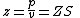Distinction has to be made between:
• the characteristic acoustic impedance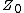of a medium, usually air (compare with characteristic impedance
Characteristic impedance
The characteristic impedance or surge impedance of a uniform transmission line, usually written Z_0, is the ratio of the amplitudes of a single pair of voltage and current waves propagating along the line in the absence of reflections. The SI unit of characteristic impedance is the ohm...

in transmission line
Transmission line
In communications and electronic engineering, a transmission line is a specialized cable designed to carry alternating current of radio frequency, that is, currents with a frequency high enough that its wave nature must be taken into account...

s).
• the impedance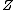of an acoustic component, like a wave conductor, a resonance chamber, a muffler or an organ pipe.

## Characteristic acoustic impedance

Characteristic acoustic impedance of air vs temperature at atmospheric pressure
The characteristic impedance of a medium, such as air
Earth's atmosphere
The atmosphere of Earth is a layer of gases surrounding the planet Earth that is retained by Earth's gravity. The atmosphere protects life on Earth by absorbing ultraviolet solar radiation, warming the surface through heat retention , and reducing temperature extremes between day and night...

, rock
Rock (geology)
In geology, rock or stone is a naturally occurring solid aggregate of minerals and/or mineraloids.The Earth's outer solid layer, the lithosphere, is made of rock. In general rocks are of three types, namely, igneous, sedimentary, and metamorphic...

or water
Water
Water is a chemical substance with the chemical formula H2O. A water molecule contains one oxygen and two hydrogen atoms connected by covalent bonds. Water is a liquid at ambient conditions, but it often co-exists on Earth with its solid state, ice, and gaseous state . Water also exists in a...

is a material property: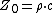where
Z0 is the characteristic acoustic impedance ([M·L–2·T−1]; N·s/m3 or Pa·s/m)
ρ
Rho
Rho is the 17th letter of the Greek alphabet. In the system of Greek numerals, it has a value of 100. It is derived from Semitic resh "head"...

is the density
Density
The mass density or density of a material is defined as its mass per unit volume. The symbol most often used for density is ρ . In some cases , density is also defined as its weight per unit volume; although, this quantity is more properly called specific weight...

of the medium ([M·L−3]; kg/m3), and
c is the longitudinal wave
Longitudinal wave
Longitudinal waves, as known as "l-waves", are waves that have the same direction of vibration as their direction of travel, which means that the movement of the medium is in the same direction as or the opposite direction to the motion of the wave. Mechanical longitudinal waves have been also...

speed or sound speed ([L·T−1]; m/s)

The characteristic impedance of air at room temperature is about 420 Pa·s/m. By comparison the sound speed and density of water are much higher, resulting in an impedance of 1.5 MPa·s/m, about 3400 times higher. This differences leads to important differences between room acoustics
Room acoustics
Room acoustics describes how sound behaves in an enclosed space.The way that sound behaves in a room can be broken up into roughly four different frequency zones:...

or atmospheric acoustics on the one hand, and underwater acoustics
Underwater acoustics
Underwater acoustics is the study of the propagation of sound in water and the interaction of the mechanical waves that constitute sound with the water and its boundaries. The water may be in the ocean, a lake or a tank. Typical frequencies associated with underwater acoustics are between 10 Hz and...

on the other.

## Specific impedance of acoustic components

The specific acoustic impedance z of an acoustic component (in N·s/m3) is the ratio of sound pressure
Sound pressure
Sound pressure or acoustic pressure is the local pressure deviation from the ambient atmospheric pressure caused by a sound wave. Sound pressure can be measured using a microphone in air and a hydrophone in water...

p to particle velocity
Particle velocity
Particle velocity is the velocity v of a particle in a medium as it transmits a wave. In many cases this is a longitudinal wave of pressure as with sound, but it can also be a transverse wave as with the vibration of a taut string....

v at its connection point: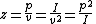where
p is the sound pressure
Sound pressure
Sound pressure or acoustic pressure is the local pressure deviation from the ambient atmospheric pressure caused by a sound wave. Sound pressure can be measured using a microphone in air and a hydrophone in water...

(N/m² or Pa),
v is the particle velocity
Particle velocity
Particle velocity is the velocity v of a particle in a medium as it transmits a wave. In many cases this is a longitudinal wave of pressure as with sound, but it can also be a transverse wave as with the vibration of a taut string....

(m/s), and
I is the sound intensity
Sound intensity
Sound intensity or acoustic intensity is defined as the sound power Pac per unit area A. The usual context is the noise measurement of sound intensity in the air at a listener's location.-Acoustic intensity:...

(W/m²)

## Complex impedance

In general, a phase relation exists between the pressure and the particle velocity. The complex impedance is defined as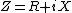where
R is the resistive part, and
X is the reactive part of the impedance

The resistive part represents the various loss mechanisms an acoustic wave experiences such as random thermal motion. For the case of propagation through a duct, wall vibrations and viscous forces at the air/wall interface (boundary layer) can also have a significant effect, especially at high frequencies for the latter. For resistive effects, energy is removed from the wave and converted into other forms. This energy is said to be 'lost from the system'.

The reactive part represents the ability of air to store the kinetic energy of the wave as potential energy since air is a compressible medium. It does so by compression and rarefaction. The electrical analogy for this is the capacitor's ability to store and dump electric charge, hence storing and releasing energy in the electric field between the capacitor plates. For reactive effects, energy is not lost from the system but converted between kinetic and potential forms.

The phase of the impedance is then given by